# Calculate the number of days between two dates using Excel

This lesson shows you how to use Excel to calculate the number of days between two dates. This might seem like a simple task, and it often is. However, in this lesson we will look at four different scenarios:

1. Calculate how many days there are between two dates, using simple calculations as well as the DATEDIF function.
2. Calculate the number of weekdays (Mon-Fri) between two dates using the NETWORKDAYS function.
3. Calculate the number of working days between two dates excluding vacation or holiday dates, also using the NETWORKDAYS function.
4. Calculate the number of business days between two dates where the weekdays may be different to Monday-Friday, using the NETWORKDAYS.INTL function.

However, we will start by looking at how Excel stores dates; this is important to understanding how Excel calculates dates in the different scenarios above.

## Excel stores dates as numbers

When you type a date into a cell in Excel, the date is actually stored as a number. This makes it possible for you to perform calculations using dates, such as subtracting one date from another to find the number of days between them.

Here are some examples of dates and the number that Excel is storing for those dates:As you'll see, this list starts at the first of January, 1900, which Excel stores as 1. From there, the dates become more recent, and the numbers stored increase.

Note also how the second of January, 1900 is stored as 2. So that means that each positive whole number refers to a specific day and date. It also means you can also perform calculations on dates as if they were numbers - because they are numbers.

There are a couple of additional things to be aware of with how Excel stores dates. These may affect how you use dates in Excel:

• Excel for Windows uses something referred to as the 1900 date system. Older versions of Excel for Mac use the 1904 date system (the number 1 represents the First of January, 1904). This is not normally an issue when transferring files between Windows and Mac computers. However, if you do have problems, you can change the settings in Excel for Mac to use the 1900 date format (or change the settings in Excel for Windows to use the 1904 date format).
• Excel can't handle dates earlier than the first of January, 1900. There are ways to work around this (as outlined in this article) but, in general, you need to remember that you can't use Excel's date system for dates earlier than 1900 (or 1904 on a Mac).
• Dates are normally expressed as whole numbers. However, it is possible to use decimal numbers to specify a specific date and time. For example, 10000.25 would be 6am on February 19, 1982 (since .25 = 25% of one day, or 6 hours).

Note that it is possible for a cell in Excel to contain text that looks like a dates. If this happens, your calculation or formula will return a #VALUE error. If this happens, check out our lesson on converting text values to dates (opens in a new tab).

## Calculate how many days there are between two dates

Now that we know that Excel stores dates as whole numbers, it is easy to use Excel to calculate the number of days between two dates - you can simply subtract one date from the other, as shown in the example below:The formula is simple - subtract the end_date from the start_date:

• =B4-B3

This example shows the same dates using two different formats. The point here is that, as long as the cells contain valid dates, the formatting you use does not affect the calculation that Excel performs.

## Use the DATEDIF function to calculate the number of days between two dates.

Another option for calculating the number of days between two dates is to use the DATEDIF function. This function was included in Excel to provide compatibility with Lotus 1-2-3 spreadsheets, and is not included in the standard list of formulas in Excel, as you can see in the following example. DATEDIF is a real function in Excel and should have appeared in the list below when we started to type =date into the formula bar.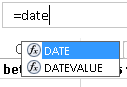To use DATEDIF to calculate the number of days between two dates, enter the following formula:

• =DATEDIF(B3,B4,"D")

This will also return the number of days between B3 and B4.

## Calculate the number of week days between two dates

If you want to calculate the number of week days (or working days) between two dates, the formula in the example above will not help. In this scenarion we can use the NETWORKDAYS function instead.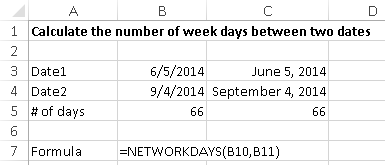The NETWORKDAYS function simply calculates the number of working days between the two dates, and ignores the weekends. The NETWORKDAYS function has this syntax:

• =NETWORKDAYS(start_date,end_date)

Note that the NETWORKDAYS function treats Saturday and Sunday as weekend days. Later, we'll see how to specify different days for the weekend.

## Calculate the number of business days between two dates, excluding holidays

You can also calculate the number of business days between two dates excluding holidays that occur during that time.

The NETWORKDAYS function can be extended with an optional parameter that specifies the holidays you want to exclude:

• NETWORKDAYS(start_date, end_date, [holidays])

The [holidays] value is a range of one or more cells that include dates which Excel should treat as holidays and exclude from the calculation.

This version of NETWORKDAYS is used in the example below: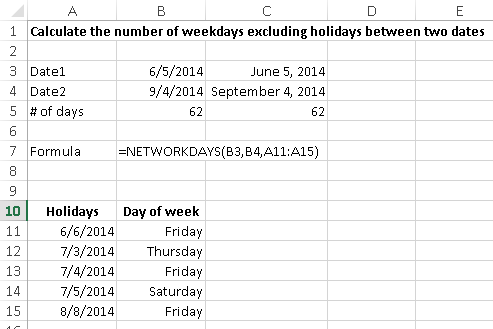As you can see, the NETWORKDAYS formula now includes a reference to A11:A15, which contains the dates to be treated as holidays:

• =NETWORKDAYS(B3,B4,A11:A15)

Note how the number of days has dropped from 66 to 62, although there are five dates included in our list of holidays. This is because the NETWORKDAYS function automatically excludes weekends from the calculation, so the fifth value on our list (which is a Saturday) has already been excluded.

## Calculate the number of week days using non-standard weekend days

What if, for your calculation, Saturday and Sunday are not considered weekend days? For example, you might work shift days which mean that your normal days off are not Saturday and Sunday.

In this case, the NETWORKDAYS won't work for you. Instead, we can use a related function, NETWORKDAYS.INTL, which looks like this:

• NETWORKDAYS.INTL(start_date, end_date, [weekend], [holidays])

Note that it is almost the same as NETWORKDAYS, but has a fourth parameter - [weekend]. Note that both [weekend] and [holidays] are optional. If you don't supply either, NETWORKDAYS.INTL functions the same as NETWORKDAYS.

The following example uses NETWORKDAYS with the [weekend] parameter included, but not the [holidays] parameter: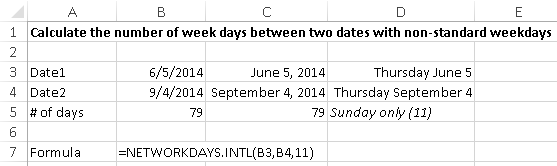This example uses the [weekend] parameter to specify that Sunday is the only weekend day of the week, for the purposes of the NETWORKDAYS.INTL calculation.

There are 14 different options for the [weekend] parameter that allow you complete control over which day(s) Excel should treat as weekends in the formula. As you can see, 11 corresponds to Sunday only.

 1 or omitted Saturday, Sunday 2 Sunday, Monday 3 Monday, Tuesday 4 Tuesday, Wednesday 5 Wednesday, Thursday 6 Thursday, Friday 7 Friday, Saturday 11 Sunday only 12 Monday only 13 Tuesday only 14 Wednesday only 15 Thursday only 16 Friday only 17 Saturday only

Fortunately, you don't have to remember this list if you're using Excel 2010 or Excel 2013 since Excel will automatically show you a list of the options available to you as you type the NETWORKDAYS.INTL function into your formula, as shown below. Unfortunately for Mac users, Excel 2011 for Mac doesn't have this feature.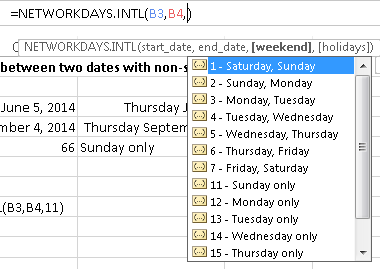## Our Comment Policy.

Add a comment to this lesson

### Calculating workdays between dates excluding holidays

OK I need some help here. I have been trying to figure this one out and cant seem to get it. I need to calculate the time is takes for one of my agents to close a ticket that has been assigned to them. This needs to exclude holidays and weekends. I want it to use the time stamp as well so it should show as 5.75 and not just 6 or 5.

My dates and times look like this:
Column O (opened) Column P (Closed)
2/27/2015 4:34:00 AM 3/10/2015 5:45:00 PM

(The time and date are in the same cell)

Here are the formulas that I have tried but none of them seem to be getting it right.

=(NETWORKDAYS(O2,P2)-1)*5/12+MOD(P2,1)-MOD(O2,1)
=NETWORKDAYS(O2,P2,Holidays!\$A\$2:\$A\$14)
=NETWORKDAYS(O2,P2)-1

Can anyone help??

### Suggested solution - calculating work days and times

Hi Marc

Here's a formula that should do the job for you:

=NETWORKDAYS(O2,P2)-2+(1-MOD(O2,1))+MOD(P2,1)

Here's what it does:

1. Finds the working days between February 27 and March 10. This returns 8 days, including those dates.
2. Subtracts 2, since two of the days were not whole days.
3. Adds back the percentage of the first day that elapsed after the ticket was opened (from 4:34am to midnight).
4. Adds back the percentage of the last day that elapsed before the ticket was closed (from midnight to 5:45pm)

The result it gives is 7.549 days.

Note that this formula will only exclude weekends. You'll need to use NETWORKDAYS.INTL function, as explained above, to exclude holidays as well.

The use of the MOD function to work out the time component of a date/time value in Excel is explained in more detail in our lesson on calculating the current date and time in Excel.

Regards

David

### How to calculate Start day + NET workdays to get the end day

Hi,
I am trying to create a ghast chart with Start date + Fixed days to accomplish that task and automatically calculate the end date by formula.

Start date: 04/24/2015
Days required for that task: 2 variable for entry
End date: 04/27/2015
(calendar days = 4, work days=2 will be automatically calculate by the formula)
Is there an easy way to accomplish that? Thanks in advance.

### Excluding weekends and 8 business hours

I am in need of some help... I am working on a NETWORKDAYS.INTL formula that should exclude weekends, holidays and cycle time of 8 business hours. The team works 8-5 mon-fri. This is what I have so far:

These 2 formulas counts the Networkdays but doesn't consider the Exact Time with the Hours

Days: =(IF(ISBLANK(C3),"",IF(ISBLANK(D3),(NETWORKDAYS(C3,TODAY())),IF(ISNUMBER(C3)+ISNUMBER(D3),(NETWORKDAYS(C3,D3))))))

Hours: =(IF(ISBLANK(C3),"",IF(ISBLANK(D3),(NETWORKDAYS(C3,TODAY())),IF(ISNUMBER(C3)+ISNUMBER(D3),(NETWORKDAYS(C3,D3))))))*24

These 2 formulas counts Networdays and factors in the Exact Time with Hours
Days: =(IF(D11="",NETWORKDAYS(C11,NOW()),NETWORKDAYS(C11,D11))-1-MOD(C11,1)+MOD(D11,1))
Hours: =(IF(D11="",NETWORKDAYS(C11,NOW()),NETWORKDAYS(C11,D11))-1-MOD(C11,1)+MOD(D11,1))*24

These 2 logics shows weekends not omitted from results and factors in the Exact Time with Hours

How would the formula be adjust? or re-written

Thanks

### number of years,months,days

Hi there, I would like to know how can I calculate the number of years/months/days in between to date .
in summery I would like to see the end result as a factor of year,month and day. example 2years,7months,3days or like 2,7,3

### Try this....

You could try this formula, where the start date is in A1 and the end date is in B1:

=YEAR(B1)-YEAR(A1)&" Years "&INT(MOD(B1-A1,365)-(INT((B1-60)/1461)-INT((A1-60)/1461)))&" Days "&HOUR(MOD(B1-A1,1))&" Hours "&MINUTE(MOD(B1-A1,1))&" Minutes"

Here's a breakdown so you can try each element of the formula as standalone formulas:

• YEAR(B1)-YEAR(A1) << calculates the number of years between the two dates
• INT(MOD(B1-A1,365)-(INT((B1-60)/1461)-INT((A1-60)/1461))) << calculates the number of days and adjusts for the number of leap years between the two dates. Assumes the dates are within 100 years of each other and will be 1 day out if they are more than 100 years apart.
• HOUR(MOD(B1-A1,1)) << calculates the number of hours
• MINUTE(MOD(B1-A1,1)) << calculates the number of minutes

Hopefully this gives you what you need. If you don't want to include the number of minutes, you can simply remove that part of the formula.

Regards

David

### Subtracting a fix number of days from a date, excluding weekends

Hi.
What is the formula to subtract fix amount of days from a date, excluding Jewish weekends (Friday, Saturday).
For example: May 10 2015 minus 2 days = May 6 2015.
Thanks
Moshe

### Date Calculation in MS Excel

Hi,

In MS Excel 2007:
How can I calculate total days required to do a job considering the below conditions:
> if the start date of the job is 01-Jan-15 (for example)
> End Date: 30-jun-15 (for example)
> only one day (Friday) as a weekly holiday
> other govt. holiday (as per govt. circular mentioning somewhere in the sheet manually)?

### networkdays function

Thanks for the lessons. However, I am trying to use the networkdays function without having to have a list of the public holidays. Is there a way of having the public holidays built into the formula, instead of having it listed separately as part of the sheet? I hope I make sense.

Regards,

### calculate to number of day

A B C
1 DATE IN Date OUT Number of Day
2 24-Nov-14 28-Jan-16 Total days

please tell me formula who can calculate the day A2 B2

### I want to exclude only first

I want to exclude only first & second saturday & sunday from date by using network days or other formula

### Calculate difference between two times/dates including weekends

Hi There,

I have tried to find any formulas that take into account a 7 day working week but include a holiday listing. Do you know if this can be done? If so, I am happy to provide some data to show what I want to get. If not, then I will just have to plod along with what I have so far.